Anúncio

# Ratio Analysis

30 de Mar de 2021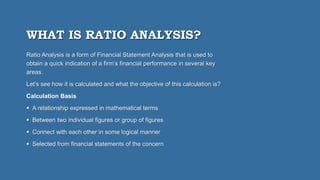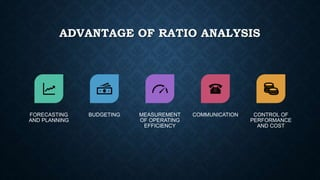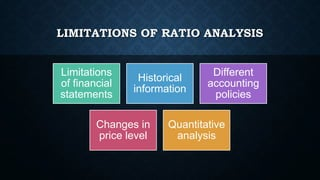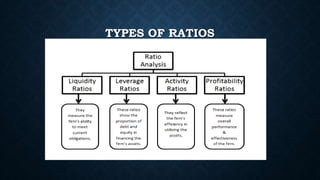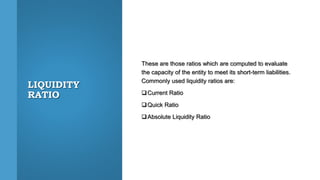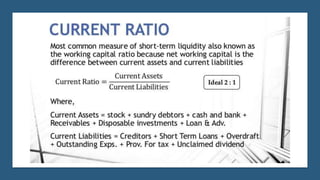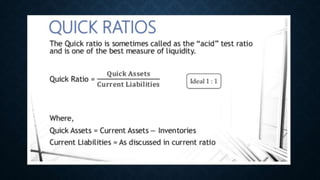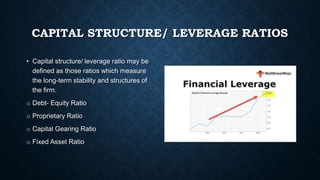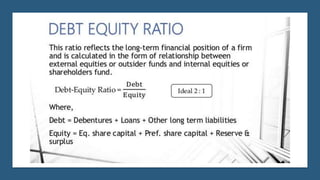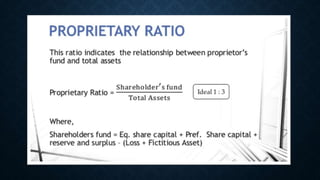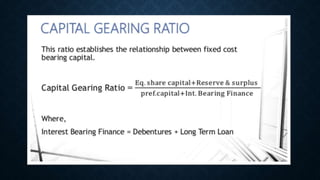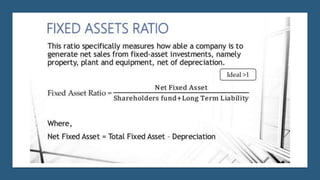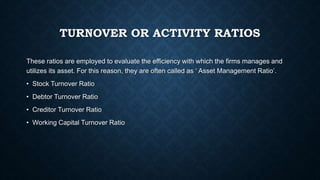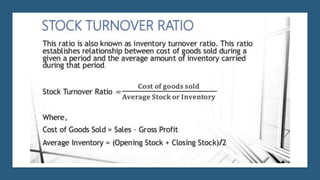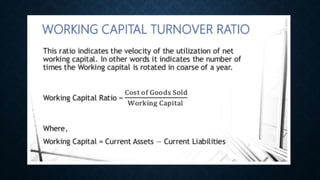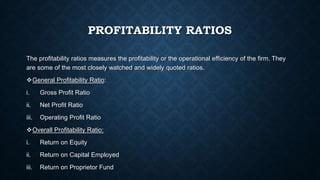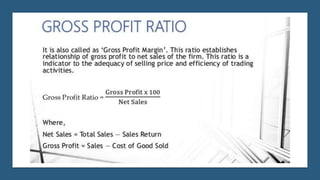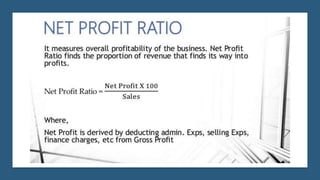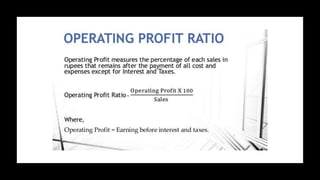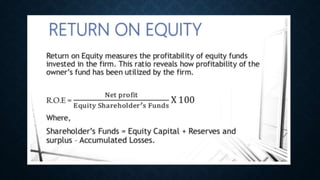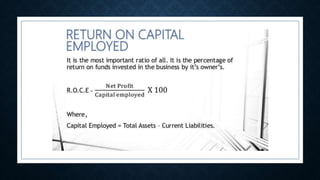1 de 28
Anúncio

### Ratio Analysis

1. RATIO ANALYSIS -BY DIVANSHU SACHDEVA, RIDDHI DEB ANINDYA DAS, AYAN SHAHI ANSHIKA GOEL & MREEDU SINGH
2. WHAT IS RATIO ANALYSIS? Ratio Analysis is a form of Financial Statement Analysis that is used to obtain a quick indication of a firm’s financial performance in several key areas. Let’s see how it is calculated and what the objective of this calculation is? Calculation Basis  A relationship expressed in mathematical terms  Between two individual figures or group of figures  Connect with each other in some logical manner  Selected from financial statements of the concern
3. OBJECTIVE • Objective for financial ratios is that all the stakeholders (owners, investors, lenders, employees, etc.) can draw conclusion about the: Performance (present, past & future) Strength and weakness of a firm Can take decisions in relation to firm
4. ADVANTAGE OF RATIO ANALYSIS FORECASTING AND PLANNING BUDGETING MEASUREMENT OF OPERATING EFFICIENCY COMMUNICATION CONTROL OF PERFORMANCE AND COST
5. LIMITATIONS OF RATIO ANALYSIS Limitations of financial statements Historical information Different accounting policies Changes in price level Quantitative analysis
6. TYPES OF RATIOS
7. LIQUIDITY RATIO These are those ratios which are computed to evaluate the capacity of the entity to meet its short-term liabilities. Commonly used liquidity ratios are: Current Ratio Quick Ratio Absolute Liquidity Ratio
8. CAPITAL STRUCTURE/ LEVERAGE RATIOS • Capital structure/ leverage ratio may be defined as those ratios which measure the long-term stability and structures of the firm. o Debt- Equity Ratio o Proprietary Ratio o Capital Gearing Ratio o Fixed Asset Ratio
9. TURNOVER OR ACTIVITY RATIOS These ratios are employed to evaluate the efficiency with which the firms manages and utilizes its asset. For this reason, they are often called as ‘ Asset Management Ratio’. • Stock Turnover Ratio • Debtor Turnover Ratio • Creditor Turnover Ratio • Working Capital Turnover Ratio
10. PROFITABILITY RATIOS The profitability ratios measures the profitability or the operational efficiency of the firm. They are some of the most closely watched and widely quoted ratios. General Profitability Ratio: i. Gross Profit Ratio ii. Net Profit Ratio iii. Operating Profit Ratio Overall Profitability Ratio: i. Return on Equity ii. Return on Capital Employed iii. Return on Proprietor Fund
11. THANK YOU!
Anúncio